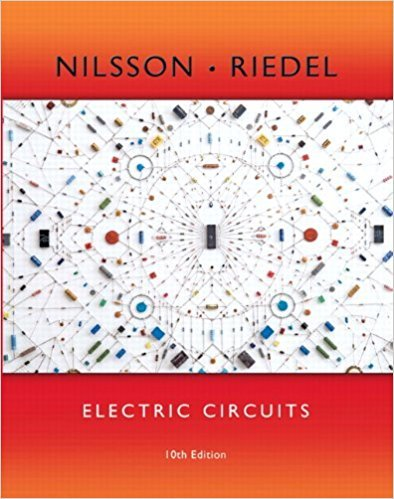×
×

# Solutions for Chapter 9.11: The Ideal Transformer## Full solutions for Electric Circuits | 10th Edition

ISBN: 9780133760033Solutions for Chapter 9.11: The Ideal Transformer

Solutions for Chapter 9.11
4 5 0 352 Reviews
31
1
##### ISBN: 9780133760033

Electric Circuits was written by and is associated to the ISBN: 9780133760033. This expansive textbook survival guide covers the following chapters and their solutions. Since 1 problems in chapter 9.11: The Ideal Transformer have been answered, more than 15026 students have viewed full step-by-step solutions from this chapter. Chapter 9.11: The Ideal Transformer includes 1 full step-by-step solutions. This textbook survival guide was created for the textbook: Electric Circuits, edition: 10.

Key Engineering and Tech Terms and definitions covered in this textbook
×